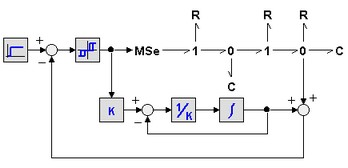﻿ 20-sim webhelp > Modeling Tutorial > Bond Graphs > Bonds and Signals

Bonds and Signals

Bonds Graph models are useful when describing systems with powerflow. In mechatronic systems these systems are usually coupled to systems that handle (powerless) signals. These systems are more conveniently described by block diagrams. Bond graphs can be combined with block diagrams. The coupling can be performed by special submodels.

Sensors

In general a sensor measures the flow, the effort, the integral of the flow or the integral of the effort. Sensors can be found in the 20-sim bond graph library.

 EffortSensor FlowSensor Psensor Qsensor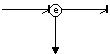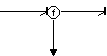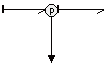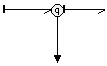measures effort measures flow measures integral off effort measures integral of flow

Junctions

In 20-sim 1 junctions have a signal output which is equal to the flow. From a 1 junction a signal can be drawn which can be used as input for a block diagram. In a similar way an effort signal can be drawn from a 0 junction.

 0 junction 1 junction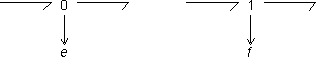Modulated Elements

The result of a block diagram can be converted into power by means of a generator. In a bond graph model, this can be done by connecting a signal to modulated source elements. These elements convert input signals into efforts or flows. Other elements that use a signal input are modulated transformers and modulated gyrators.

Example

An example model where a bond graph and a block diagram are coupled is shown in the picture below. As can be seen an effort signal is measured at the 0 junction. In the block diagram part it is processed and fed back into the bond graph at the modulated effort source.